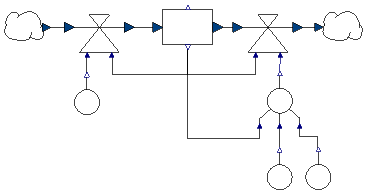# Population models

• Basic model:
• stock describes size N of population
• number of births and deaths proportional to N
• G = g N
T = t N
• rates g, t in ConstantConverter blocks
• products with Mult2Flow blocks
• model population1
•• result for g = 0.03, t = 0.01: exponential growth
• Limited growth:
• scarcity of resources → death rate grows for large population
• approach: death rate proportional to N
• t = (N/Nb) tb
• implement equation with MultPower3Converter
• out = in1k1 * in2k2 * in3k3
• N: in1 = stock.out1, k1 = 1
• tb: in2 = 0.01 (coming from ConstantConverter), k2 = 1
• Nb: in3 = 50 (coming from ConstantConverter), k3 = -1
• complete model population2
•• result
• change plot range with plot setup to [0, 150]
•• Fixed capacity:
• changes in population3
• death rate stays constant for small N
• N has upper limit Nk
• idea
•• no predefined converter for this formula
• replace MultPower3Converter by CapacityConverter using Modelica code
• block CapacityConverter
extends SystemDynamics.Interfaces.GenericConverter3;
equation
out1 = in2/(1 - in1/in3);
end CapacityConverter;
• create new component
• in OpenModelica: File/New/New Modelica Class
• Name: CapacityConverter
• Extends: SystemDynamics.Interfaces.GenericConverter3
• Insert in class: SystemDynamicsExamples.AuxComponents
• set Nk = 225
• result
• similar to previous version
•• "curve fits data" does not imply "model mechanism is correct"!